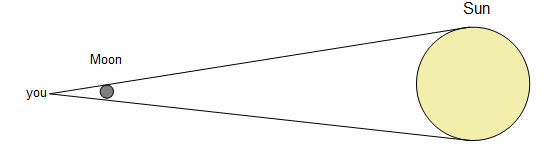# Question about angles and triangles I thinkg

pbody
1. How does the moon's diameter compare with the distance between Earth and the Moon

Tape a small coin, such as a dime to a window and view it with one eye so that it just blocks out the full Moon. This occurs when your eye is about 110 coin diameters away. Then the ratio of coin diameter/ coin distance is about 1/110. Geometrical reasoning of similar triangles shows this is also the ratio of Moon diameter/Moon distance. So the distance to the Moon is 110 times the Moon's diameter. The early Greeks knew this. Aristarchus's measurement of the Moon's diameter was all that was needed to calculate the Earth - Moon distance. So the early Greeks knew both the size of the Moon and its distance from Earth.

2. moon diameter is 1 and the moon distance is 110 1/110

3. I understand that looking at the moon it is a total of 1 moon diameter and the distance is 110 moons away.

but I don't understand at the same time where and how this is theasable. How would you correctly get that measurement and why. I know this man Aritarchus didn't have 110 moons so I am assuming he used geometry but how did he decide on the angle, or what ever.

I don't know if I am making sense.

pbody
Than it also states that you can use the same method with the sun, which is 1/110. I do not understand basically because I am assuming if you did this with Jupiter there would be a lot more Jupiters than 110.

Homework Helper
Gold Member
The greeks new the bit about the coin. eg that a coin held 110 diameters away blocked out the moon. That's an easy experiment to do.

They also understood the properties of "similar triangles"... Read down to and including "Example 27" , Don't worry there aren't 27 examples to read!..

http://www.mathsteacher.com.au/year9/ch13_geometry/08_similar/similar.htm

The angle subtended by the coin and moon are the same (0.54°) so...

Coin Diameter/Coin distance = Moon Diameter/Moon Distance

So all they needed was the moon diameter and they could calculate it's distance.

Then along came Aritarchus and he worked out how to measure the diameter..

Homework Helper
Gold Member
Than it also states that you can use the same method with the sun, which is 1/110. I do not understand basically because I am assuming if you did this with Jupiter there would be a lot more Jupiters than 110.

Thats because by chance the angle subtended by the moon and sun is very similar..

0.54 degrees for the moon
0.52 degrees for the sun

See..

http://www.mathopenref.com/subtend.html

or put it another way...The moon just happens to be the right size and distance away so that it appears the same size as the much bigger and further away sun.eg by pure fluke...

Moon diameter/moon distance ≈ sun diameter/sun distance

It does not work for Jupiter because Jupiter is the wrong size and distance.

pbody
I will be back I have to go to the store and get a protractor I don't know where mine is and my desk is an absolute mess!

pbody
In theory couldn't you perceive let's still use Jupiter as a similar angle comparison to the moon? couldn't you have Jupiter diameter and Jupiter distance which of course would be more than the moon and sun distance of 110. but depending on how you look at it would you be able to contort it to the angle of which you want? I know we started measuring distance in light years over angular measurements but I am a little confused.

pbody
In theory couldn't you perceive let's still use Jupiter as a similar angle comparison to the moon? couldn't you have Jupiter diameter and Jupiter distance which of course would be more than the moon and sun distance of 110. but depending on how you look at it would you be able to contort it to the angle of which you want? I know we started measuring distance in light years over angular measurements but I am a little confused.

I am not trying to be smart I am just trying to understand to the best of my capabilities.

*wait - I just thought of this did they do the measurements during a solar eclipse, because if they did that would make sense I am looking at the diagram and everything seems to be aligned as if there was and eclipse or something.